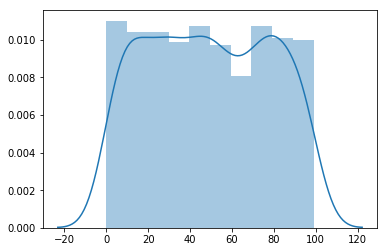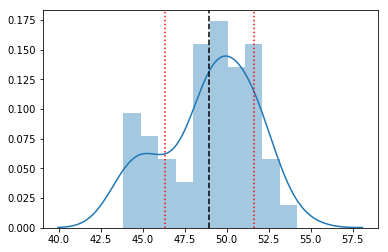# verifying_central_limit_theorem

## Verifying Central Limit Theorem¶¶

The Central Limit Theorem states that the sampling distribution of the sampling means approaches a normal distribution as the sample size gets larger — no matter what the shape of the population distribution. This fact holds especially true for sample sizes over 30. All this is saying is that as you take more samples, especially large ones, your graph of the sample means will look more like a normal distribution.

In :
import pandas as pd
import numpy as np
import matplotlib.pyplot as plt
import seaborn as sns
%matplotlib inline


### Generate 1k random integers¶¶

Let us use NumPy to generate 1000 random integers between the range 0-100. Our objective is to calculate the population mean and verify if the mean obtained using CLT comes close to population mean.

In :
rand_1k = np.random.randint(0,100,1000)

In :
rand_1k.size

Out:
1000
In :
sns.distplot(rand_1k)

/Users/atma6951/anaconda3/envs/pychakras/lib/python3.6/site-packages/matplotlib/axes/_axes.py:6462: UserWarning: The 'normed' kwarg is deprecated, and has been replaced by the 'density' kwarg.
warnings.warn("The 'normed' kwarg is deprecated, and has been "

Out:
<matplotlib.axes._subplots.AxesSubplot at 0x1a19f2c048>Thus the population follows a uniform distribution, not a normal distribution. Still, we will see the distribution of our means will follow a normal distribution.

#### Calculate population mean¶¶

In :
np.mean(rand_1k)

Out:
48.826

#### Try out creating a subset and finding its mean¶¶

In :
subset_100 = np.random.choice(rand_1k, size=100, replace=False)
subset_100.size

Out:
100
In :
np.mean(subset_100)

Out:
43.2

The mean of this subset of 100 integers is 43.2. Not close enough.

### Apply CLT.¶¶

We will generate 50 samples with 100 items each and find their means.

In :
# generate 50 random samples of size 100 each
subset_means = []
for i in range(0,50):
current_subset = np.random.choice(rand_1k, size=100, replace=False)
subset_means.append(np.mean(current_subset))


Calculate the mean of means (its meta :))

In :
clt_mean = np.mean(subset_means)
clt_mean

Out:
48.9768

Calculate the SD of the means

In :
subset_sd = np.std(subset_means)
subset_sd

Out:
2.657234983963594
In :
ax = sns.distplot(subset_means, bins=10)
# draw mean in black
ax.axvline(clt_mean, color='black', linestyle='dashed')

# draw mean +- 1 SD
ax.axvline(clt_mean + subset_sd, color='red', linestyle='dotted')
ax.axvline(clt_mean - subset_sd, color='red', linestyle='dotted')

/Users/atma6951/anaconda3/envs/pychakras/lib/python3.6/site-packages/matplotlib/axes/_axes.py:6462: UserWarning: The 'normed' kwarg is deprecated, and has been replaced by the 'density' kwarg.
warnings.warn("The 'normed' kwarg is deprecated, and has been "

Out:
<matplotlib.lines.Line2D at 0x1a1ac5f908>Difference between mean of means and the population mean

In :
np.mean(rand_1k) - clt_mean

Out:
-0.15079999999999671